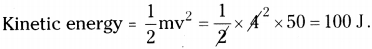AP State Syllabus AP Board 9th Class Physical Science Important Questions Chapter 10 Work and Energy

## AP State Syllabus 9th Class Physical Science Important Questions 10th Lesson Work and Energy

### 9th Class Physical Science 10th Lesson Work and Energy 1 Mark Important Questions and Answers

Question 1.
What type of energies are possessed by the objects shown in below figures.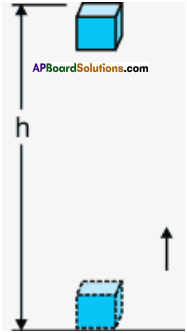i) Potential energy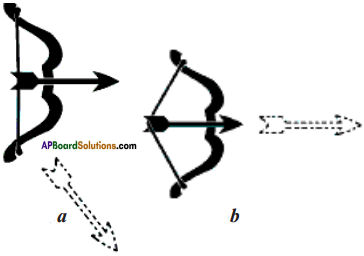ii) Kinetic energy

### 9th Class Physical Science 10th Lesson Work and Energy 2 Marks Important Questions and Answers

Question 1.
According to the scientific concept of the work, two conditions need to be satisfied in order to say that work has been done.
i. A force should act on the object.
ii. The object must be displaced or there must be change in position of the object. Now complete the table given below.

 Situation been done or not Whether work has Reason to say that work has been done or not A boy lifts his bag from the ground A girl is trying to push a huge rock

 Situation been done or not Whether work has Reason to say that work has been done or not A boy lifts his bag from the ground done 1) Force acted on the bag. 2) Bag moved from the ground. A girl is trying to push a huge rock not done 1) Force acted on the huge rock. 2) Rock not moved.

### 9th Class Physical Science 10th Lesson Work and Energy 4 Marks Important Questions and Answers

Question 1.
Write the differences between potential energy and kinetic energy.
(OR)
Compare and differentiate between political energy and kinetic energy.

 Kinetic energy Potential energy 1) K.E is the kind of energy present in a body due to the property of its motion. 1) P.E is the type of energy present in a body due to the property of its state. 2) It can be easily transferred from one body to another. 2) It is not transferable. 3) K.E = ½ mv2 3) PE = mgh 4) Flowing water is example of K.E. 4) Water present at the top of hill is an example of P.E.

### 9th Class Physical Science 10th Lesson Work and Energy Important Questions and Answers

9th Class Physical Science 10th Lesson Work and Energy 1 Mark Important Questions and Answers

Question 1.
What is meant by one joule work?
1 joule work is the amount of work done on an object when a force of 1 Newton displaces it by 1 m along the line of action of the force.Question 2.
What happens to the speed of a ball while it moves up with an initial velocity?
As the ball moves up, the gravitational force acts against to the direction of motion of the object. Hence its velocity slowly decreases.

Question 3.
When a ball is moving up with an initial velocity, what will be its speed at its maximum height?
The speed of a ball moving up is zero at its maximum height.

Question 4.
What happens to the speed of the ball during its downward motion?
The speed of the ball during its downwards motion will increase gradually.

Question 5.
Define energy.
Energy is defined as the capacity to do a work.Question 6.
Why does a person get tired standing at a place for long time?
Though the person standing is not doing any work externally a lot of work is being done inside the body.

The muscles of the body become stretched when he stands for long time and heart has to pump more blood to muscles. This leads to loss of energy inside the body and hence he gets tired.

Question 7.
What is kinetic energy?
The energy possessed by an object due to its motion is called kinetic energy.

Question 8.
Write a formula to measure kinetic energy.
KE = $$\frac{1}{2}$$mv²

Question 9.
Define potential energy.
The energy possessed by an object because of its position or shape is called its potential energy.Question 10.
Write a formula to calculate potential energy.
Potential energy PE = mgh.

Question 11.
What is conservation of energy?
The law of conservation of energy states that the energy can neither be created nor destroyed. It can only be changed from one form to another.

Question 12.
A battery lights a bulb. Describe the energy changes involved in the process?
Chemical energy is converted into heat energy then light energy.

Question 13.
A mass of 10 kg at a point A on the table is moved to a point B. If the line joining A & B is horizontal, what is the work done on the object by gravitational force? Explain your answer.
The work done on the object by gravitational force is zero, since the angle between force and motion of object is zero.

Question 14.
A person holds a bundle of hay over his head of 30 minutes and get tired. Has he done some work or not? Justify your answer.
There is no displacement. So no work is done on the object. (W = F x s = F x 0 = 0)Question 15.
Expand OTE.
OTE means – Ocean Thermal Energy.

Question 16.
Expand OTEC.
OTEC means – Ocean Thermal Energy Conversion.

Question 17.
What is ‘Biomass’?
The material contained in the bodies of plants and animals is Called ‘Biomass’.

Question 18.
What is Nuclear reaction?
The physical reaction which involves changes in nucleus of an atom is called “Nuclear reaction”.Question 19.
What is Nuclear Energy?
The energy released during a nuclear reaction is called nuclear energy.

9th Class Physical Science 10th Lesson Work and Energy 2 Marks Important Questions and Answers

Question 1.
Define power and mention its units.
Power is defined as the rate of doing work or rate of transfer of energy.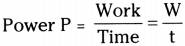The unit of power is ‘Watt’ and denoted by the symbol ‘W’.

Question 2.
What is the meaning of one joule?
One joule is the amount of work done on an object when a force of 1 Newton displaces it by 1 m along the line of action of the force.
If F = 1N, s = 1 m then the work done
W = F × s = 1 N × 1 m = 1 N-m = 1 JouleQuestion 3.
What is the meaning of one watt?
1 watt is the power of an object, which does work at the rate of one joule per second.
If W = 1J, t = 1 sec then,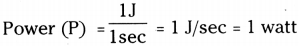Question 4.
Explain work through a diagram.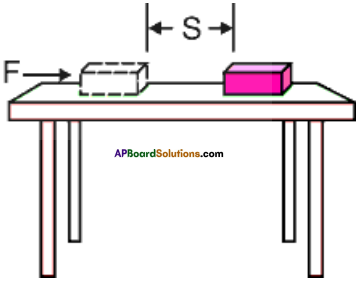1. Suppose a constant force (F) acts on an object and is moved through a distance (s) along the direction of the force, as shown in figure.
2. Then the work done W = F × s

Question 5.
Certain force acting on a 20 kg mass changes it velocity from 5 ms-1 to 2 ms-1. Calculate the work done by the force?
Mass (m) = 20 kg
Initial velocity (u) = 5 m/s
Final velocity (v) = 2 m/s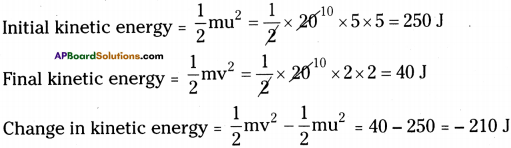Change in kinetic energy stored as work done is – 210 J

Question 6.
The potential energy of a freely falling object decreases progressively. Does the violate the law of conservation of energy? Why?
No, it is not violating law of conservation of energy because when freely falling object moves towards ground its speed increases due to gravitational force so potential energy is converted into kinetic energy.

Question 7.
Calculate the work required to be done to stop a car of 1500 kg moving at a velocity of 60 km/hr.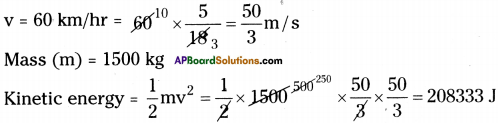∴ The work done to stop the moving car = 208333 J

Question 8.
An object of mass m is moving with a constant velocity v. tlow much work should be done on the object in order to bring the object to rest?
Mass of the object = m, Velocity of the object = v, Kinetic energy = $$\frac{1}{2}$$ mv².
The work done to bring the object to rest is stored in the from of kinetic energy.
∴ Work done = $$\frac{1}{2}$$ mv²

Question 9.
In each of the following a force, F is acting on an object of mass, m. The direction of displacement is from west to east shown by the long arrow. Observe the diagrams carefully and state whether the work done by the force is negative, positive or zero.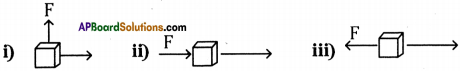i) In the first case force is acting perpendicular to the displacement of the object. So the work done by the force is zero.
ii) In the second case force is acting in the direction of displacement. So work done
by the force is positive.
iii) In the third case force and displacement opposite to each oth£r. So the work done is negative. –

Question 10.
What is Nuclear fission?
1) The process in which heavy nucleus of a radioactive atom splits up into smaller nuclei when bambared with low energy neutrons is called nuclear fission.
2) Ex : 235U92 + 1n0139Ba56 + 94Kr36 + 31n0 + EnergyQuestion 11.
What is nuclear fusion?
1) The process which two nuclei of light elements combine to form a heavy nucleus is called nuclear fusion.
2) Ex : 2H1 + 2H1 → 3He2 + 1n0 + Energy.

Question 12.
What is Ocean Thermal Energy?

1. Heat from the sun is absorbed by the water on the surface of ocean, but at deeper levels of ocean, the temperature is very less.
2. So, there is temperature difference between the water “at the surface of ocean” and at “deep levels”.
3. This difference in temperature is called Ocean Thermal Energy.

9th Class Physical Science 10th Lesson Work and Energy 4 Marks Important Questions and Answers

Question 1.
Explain negative value of work done.

1. Suppose a ball is moving on a plain ground, will get stopped after sometime due to frictional force acting on it in opposite direction.2. If the force acting on an object and displacement are in opposite directions then the work done by the force is taken as negative.
W = – F × s
3. If the work done has negative value, the body on which the work has been done loses energy.

Question 2.
Look at the activities listed below. Reason out whether or not, work is done in the light of your understanding of the term work.
1. Suma is swimming in a pond.
2. A donkey is carrying a load on its back.
3. A wind-mill lifting water from a well.
4. A green plant is carrying out photosynthesis.
5. An engine is pulling a train.
6. Food grains are getting dried in the sun.
7. A sail boat is moving due to wind energy.

1. Work is done because force is applied and the person is moved.
2. No work is done the object is not moved in the direction of force.
3. Work is done against gravitational force thrift water.
4. No work is done
5. Work is done since the engine is pulling the train.
6. No work is done.
7. Work isjdone because sail boat is moving in the direction of wind energy.Question 3.
An object of mass 4 kg is raised to height of 5 m above the ground. What is its potential energy? If the object is allowed to fall, find its kinetic energy when it is half way down.